Courses

# Height and Distance, Quantitative Aptitude, Civil Service Examination, RPSC UPSC Notes | EduRev

## RAS RPSC Prelims Preparation - Notes, Study Material & Tests

Created by: Notes Wala

## UPSC : Height and Distance, Quantitative Aptitude, Civil Service Examination, RPSC UPSC Notes | EduRev

The document Height and Distance, Quantitative Aptitude, Civil Service Examination, RPSC UPSC Notes | EduRev is a part of the UPSC Course RAS RPSC Prelims Preparation - Notes, Study Material & Tests.
All you need of UPSC at this link: UPSC

This topic has many practical application in day to day life. In engineering stage it is used in surveying. The basic purpose is to find the unknown variables by observing the angle of the line of sight. This is done by using some the fact that in a right angled triangle the ratio of any two sides is a function of the angle between them. From exam point of view this is one of the more tough sections and tedious to some extent. So an aspirant must thoroughly solve all the questions given here.

Important Formulas

1. Trigonometric Basics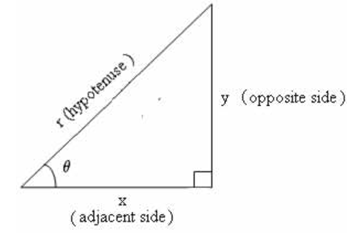sinθ = opposite side/hypotenuse=y/r

cosecθ = hypotenuse/opposite side=r/y

From Pythagorean theorem, x2+y2=r2 for the right angled triangle mentioned above

2. Basic Trigonometric Values

 θin degrees θin radians sinθ Cosθ tanθ 0° 0 0 1 0 30° π/6 1/2 3/√2 1/√2 45° π/4 1/√2 1/√2 1 60° π/3 3/√2 1/2 √2 90° π/2 1 0 Not defined

3. Trigonometric Formulas

Degrees to Radians and vice versa

Trigonometry - Quotient Formulas

tanθ=sinθ/cosθ

cotθ=cosθ/sinθ

Trigonometry - Reciprocal Formulas

cosecθ=1/sinθ

secθ=1/cosθ

cotθ=1/tanθ

Trigonometry - Pythagorean Formulas

sin2θ+cos2θ=1

sec2θ−tan2θ=1

cosec2θ−cot2θ=1

4. Angle of Elevation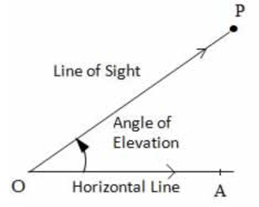Suppose a man from a point O looks up at an object P, placed above the level of his eye. Then, angle of elevation is the angle between the horizontal and the line from the object to the observer's eye (the line of sight).

i.e., angle of elevation =  ∠AOP

5. Angle of Depression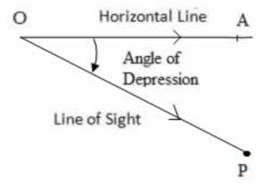Suppose a man from a point O looks down at an object P, placed below the level of his eye. Then, angle of depression is the angle between the horizontal and the observer's line of sight

i.e., angle of depression = ∠AOP

6. Angle Bisector Theorem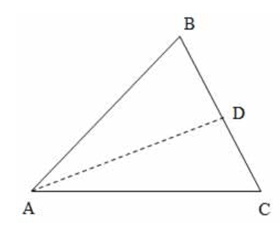Consider a triangle ABC as shown above. Let the angle bisector of angle A intersect side BC at a point D. Then BD/DC=AB/AC

(Note that an angle bisector divides the angle into two angles with equal measures.

7. Few Important Values to memorize

√2=1.414, √3=1.732, √5=2.236

Solved Examples

 1. The angle of elevation of a ladder leaning against a wall is 60º and the foot of the ladder is 12.4 m away from the wall. The length of the ladder is: A. 14.8 m B. 6.2 m C. 12.4 m D. 24.8 m

Explanation :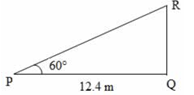Consider the diagram shown above where PR represents the ladder and RQ represents the wall.

cos 60° = PQ/PR

1/2=12.4/PR

PR=2×12.4=24.8 m

 2. From a point P on a level ground, the angle of elevation of the top tower is 30º. If the tower is 200 m high, the distance of point P from the foot of the tower is: A. 346 m B. 400 m C. 312 m D. 298 m

Explanation :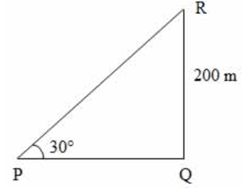tan 30°=RQ/PQ

1/√3=200/PQ

PQ=200√3=200×1.73=346 m

 3.The angle of elevation of the sun, when the length of the shadow of a tree is equal to the height of the tree, is: A. None of these B. 60° C. 45° D. 30°

Explanation :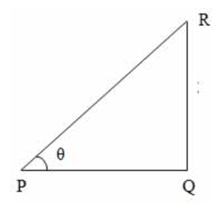Consider the diagram shown above where QR represents the tree and PQ represents its shadow

We have, QR = PQ

Let  ∠QPR = θ

tan θ = QR/PQ=1 (since QR = PQ)

=> θ = 45°

i.e., required angle of elevation = 45°

 4. An observer 2 m tall is 103√ m away from a tower. The angle of elevation from his eye to the top of the tower is 30º. The height of the tower is: A. None of these B. 12 m C. 14 m D. 10 m

Explanation :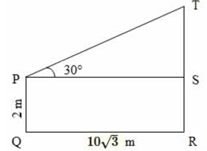SR = PQ = 2 m

PS = QR = 10√3m

tan 30°=TS/PS

1/3=TS/10√3

TS=10√3/√3=10 m

TR = TS + SR = 10 + 2 = 12 m

 5. From a tower of 80 m high, the angle of depression of a bus is 30°. How far is the bus from the tower? A. 40 m B. 138.4 m C. 46.24 m D. 160 m

Explanation :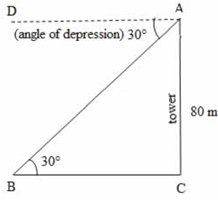Let AC be the tower and B be the position of the bus.

Then BC = the distance of the bus from the foot of the tower.

Given that height of the tower, AC = 80 m and the angle of depression, ∠DAB = 30°

∠ABC =  ∠DAB = 30° (Because DA || BC)

tan 30°=AC/BC =>tan 30°=80/BC =>BC = 80/tan 30°=80/(1/√3)=80×1.73=138.4 m

i.e., Distance of the bus from the foot of the tower = 138.4 m

 6. Find the angle of elevation of the sun when the shadow of a pole of 18 m height is 6√3 m long? A. 30° B. 60° C. 45° D. None of these

Explanation :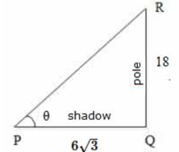Let RQ be the pole and PQ be the shadow

Given that RQ = 18 m and PQ = 6√3 m

Let the angle of elevation,  RPQ = θ

From the right   PQR,

tanθ=RQ/PQ=18/6√3=3/√3=(3×√3)/(√3×√3)=3√3/3=√3

θ=tan−1(3√)=60°

 7. A man on the top of a vertical observation tower observers a car moving at a uniform speed coming directly towards it. If it takes 8 minutes for the angle of depression to change from 30° to 45°, how soon after this will the car reach the observation tower? A. 8 min 17 second B. 10 min 57 second C. 14 min 34 second D. 12 min 23 second

Explanation :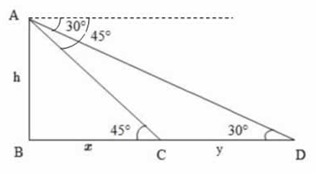Consider the diagram shown above. Let AB be the tower. Let D and C be the positions of the car

Then, ∠ADC = 30° , ∠ACB = 45°

Let AB = h, BC = x, CD = y

tan 45°=AB/BC=h/x

⇒1= h/x ⇒ h=x------(1)

tan 30°=AB/BD = AB/(BC + CD)=h/(x+y)

⇒1/√3 = h/(x+y)

⇒x + y = √3h

⇒y = √3h – x

⇒ y = √3h−h(∵ Substituted the value of x from equation 1 )

⇒ y = h(√3−1)

Given that distance y is covered in 8 minutes i.e, distance h(√3−1) is covered in 8 minutes

Time to travel distance x = Time to travel distance h (∵ Since x = h as per equation 1).

Let distance h is covered in t minutes

since distance is proportional to the time when the speed is constant, we have

h(√3−1)∝8---(A)

h∝t---(B)

(A)/(B) ⇒ h(√3−1)/h = 8/t

⇒ (√3−1) = 8/t

⇒ t = 8/(√3−1) = 8/(1.73−1) = 8/.73 = 800/73 minutes ≈10 minutes 57 seconds

 8. The top of a 15 metre high tower makes an angle of elevation of 60° with the bottom of an electronic pole and angle of elevation of 30° with the top of the pole. What is the height of the electric pole? A. 5 metres B. 8 metres C. 10 metres D. 12 metres

Explanation :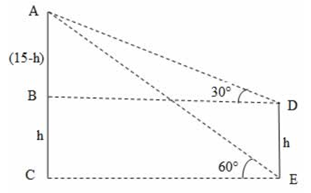Consider the diagram shown above. AC represents the tower and DE represents the pole

Given that AC = 15 m , ∠ADB = 30°, ∠AEC = 60°

Let DE = h

Then, BC = DE = h, AB = (15-h) (∵ AC=15 and BC = h), BD = CE

tan 60°=AC/CE ⇒√3=15/CE ⇒CE = 15√3--- (1)

tan 30°=AB/BD ⇒1/√3=(15−h)/BD

⇒1/√3=(15−h)/(15/√3)(∵ BD = CE and Substituted the value of CE from equation 1)

⇒(15−h)=(1/√3)×(15/√3)=15/3=5

⇒h=15−5=10 m

i.e., height of the electric pole = 10 m

 9. Two ships are sailing in the sea on the two sides of a lighthouse. The angle of elevation of the top of the lighthouse is observed from the ships are 30º and 45º respectively. If the lighthouse is 100 m high, the distance between the two ships is: A. 300 m B. 173 m C. 273 m D. 200 m

Explanation :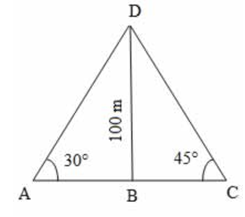Let BD be the lighthouse and A and C be the positions of the ships.
Then, BD = 100 m, ∠BAD = 30° , ∠BCD = 45°

tan 30° = BD/BA ⇒1/√3=100/BA

⇒ BA=100√3

tan 45° = BD/BC

⇒1=100/BC

⇒ BC=100

Distance between the two ships = AC = BA + BC
=100√3+100=100(√3+1)
= 100(1.73+1)
= 100×2.73=273 m

 10. From the top of a hill 100 m high, the angles of depression of the top and bottom of a pole are 30° and 60° respectively. What is the height of the pole? A. 52 m B. 50 m C. 66.67 m D. 33.33 m

Explanation :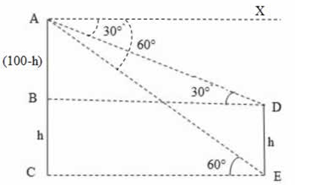Consider the diagram shown above. AC represents the hill and DE represents the pole

Given that AC = 100 m

XAE =  AEC = 60° (∵ AX || CE)

Let DE = h

Then, BC = DE = h, AB = (100-h) (∵ AC=100 and BC = h), BD = CE

tan 60°=AC/CE

⇒ √3=100/CE ⇒ CE = 100/√3--- (1)

tan 30°=AB/BD ⇒ 1/√3=(100−h)/BD

⇒1/√3=(100−h)/(100/√3)(∵ BD = CE and Substituted the value of CE from equation 1)

⇒(100−h)=1/√3×100/√3=100/3=33.33⇒h=100−33.33=66.67 m

i.e., the height of the pole = 66.67 m

Offer running on EduRev: Apply code STAYHOME200 to get INR 200 off on our premium plan EduRev Infinity!

## RAS RPSC Prelims Preparation - Notes, Study Material & Tests

109 docs|21 tests

,

,

,

,

,

,

,

,

,

,

,

,

,

,

,

,

,

,

,

,

,

,

,

,

,

,

,

,

,

,

;